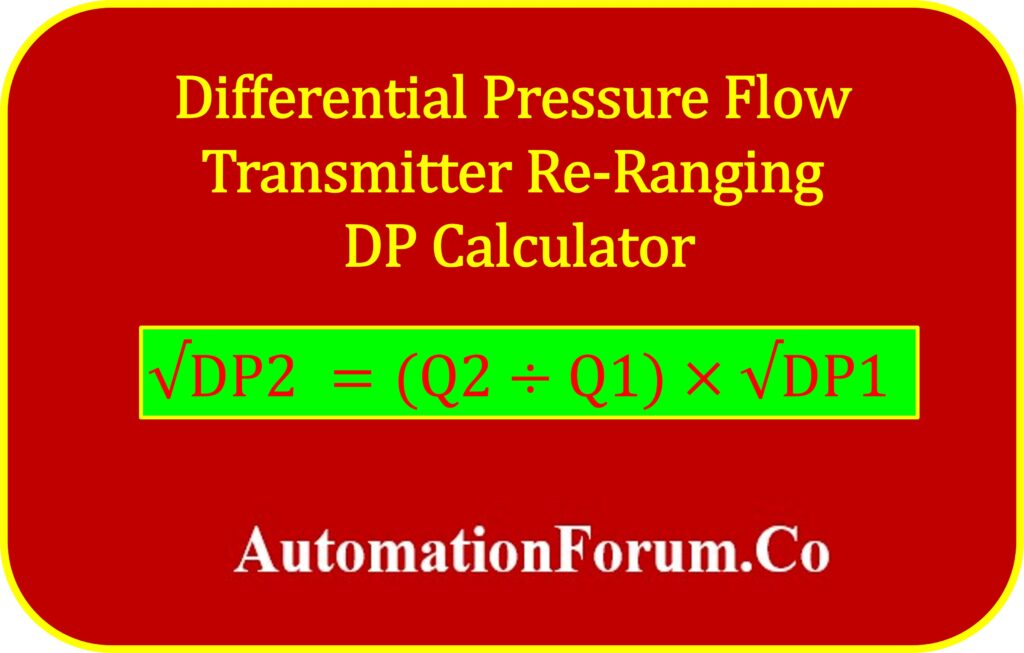# DP Flow Transmitter Re-Ranging CalculatorTo re-range a differential pressure (DP) flow transmitter in terms of differential pressure when the flow rate changes, you can use a formula that incorporates the relationship between flow rate and differential pressure. This formula allows you to determine the new differential pressure range required to accommodate a new flow rate.

## Formula for re-ranging the flow transmitter

The formula for re-ranging the differential pressure (DP2) with respect to the new flow rate (Q2) is:

Where:

Q1 is the current flow rate

DP1 is the current differential pressure range

Q2 is the new revised  flow rate,

DP2 is the new revised differential pressure range(need to be calculated)

Note:

1. The current DP and the revised DP need to use the same units, such as bars, millibars, millimeters of mercury, and so on.

2. Both the existing flow and the revised flow have to be expressed in the same units, such as m³/h or t/hr.

This calculation tool is usually utilized with orifice-based flow meters.

Typically, we calibrate our transmitter in accordance with the orifice’s datasheet. The DP flow transmitter must sometimes be re-ranged after installation if the measured DP is out of range or exceeds the maximum scale of DP.

## Example calculation

To illustrate how this formula is applied, let’s work through an example:

Let’s say you have a differential pressure flow transmitter with the following parameters:

Current differential pressure range (DP1) = 225 mBar

Current flow rate (Q1) = 100 m³/h

New revised  flow rate (Q2) = 200 m³/h

Using the formula, we can calculate the new differential pressure range (DP2) for the transmitter after re-ranging:

New revised differential pressure (DP2) = 900 mBar

Therefore, when the flow rate changes from 100 m³/h to 200 m³/h, the new differential pressure range (DP2) for the transmitter should be set and calibrated to 900 mBar.

## Re-Ranging Calculator

This below calculator is intended to determine the DP Flow transmitter revised differential pressure using the following inputs: Existing DP, Existing Flow, and Revised flow.